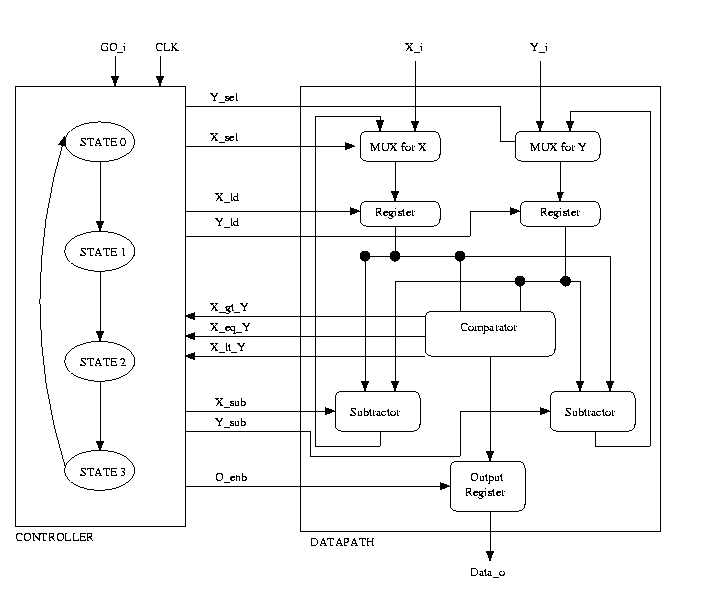FSM + D: Greatest Common Divisior

I. Introduction

The purpose of this lab is to implement a finite state machine in VHDL to calculate the Greatest Common Divisor(GCD) of 2 numbers.

The algorithim used to compute the GCD is as follows. Two numbers are compared ( x = y ?). If so the the GCD is found. If x > y, then x = x - y. The two numbers are then compared once again. If y > x, then y = y - x. The two numbers are then compared once again. Here is and example of our algorithim:

x = 10
y = 2

Is x = y? No, x > y therefore x = x - y
in our case, x = 10 - 2 = 8.

Is x = y? No, x > y therefore x = x - y
In our case, x = 8 - 2 = 6.

Is x = y? No, x > y there fore x = x - y
In our case, x = 6 - 2 = 4.

Is x = y? No, x > y therefore x = x - y
In our case, x = 4 - 2 = 2.

Is x = y? Yes, therefore the GCD of 10 and 2 is 2.

Note that 0 is not a valid input.

The design of the GCD calculator should be divided into 2 parts - a controller and a datapath. The controller is an FSM which issues commands to the datapath based on the current state and the external inputs. This can be a behavioral description. The datapath contains a netlist of functional units like multiplexors, registers, subtractors and a comparator, and hence this design is structural. The controller basically steps through the GCD algorithim shown above. If x = y, we have finished computing the GCD, and we go to the final state and assert the data output line. The Datapath does the actual GCD computation. It has the following components:

• Mux: takes 2 4-bit inputs and one select line. Based on the select line, it outputs either the 1st 4-bit number or the 2nd 4-bit number.
• Register: Takes a 4-bit input, a load signal, reset, and a clock signal. If the load signal is high and the clock is pulsed, it outputs the 4-bit number.
• Comparator: Takes 2 4-bit numbers, and assets one of 3 signals depending on whether the 1st number is less than, greater than or equal to the 2nd number.
• Subtractor: Takes 2 4-bit numbers, subtracts the smaller number from the larger.
• Output Register: Holds the GCD value. When x = y the GCD has been found and can be outputted. Because it is a register entity it should also take a clock and reset signal.Sample Structure of the Controller and Datapath

II. Procedure

implementation and simulation:

1. For an idea of how the gcd calculator might look, refer to the above figure. However, you state machine can have a different number of states, archs, and loops.
2. Create an FSM to reflect the GCD calculation process.
3. Convert the FSM to an FSM +D
4. Write the VHDL code to reflect the controller and data path.
5. Write a test bench and verify the correctness of your design using Aldec Active-VHDL